LEGACY CONTENT. If you are looking for Voteview.com, PLEASE CLICK HERE

This site is an archived version of Voteview.com archived from University of Georgia on May 23, 2017. This point-in-time capture includes all files publicly linked on Voteview.com at that time. We provide access to this content as a service to ensure that past users of Voteview.com have access to historical files. This content will remain online until at least January 1st, 2018. UCLA provides no warranty or guarantee of access to these files.

45-733 Probability and Statistics I (3rd Mini AY 1999-2000 Flex-Mode and Flex-Time)

5.1 (a)
```
Y2

1   2   3
------------
1 | 1   2   1 | 4
|           |
Y1  2 | 2   2   0 | 4
|           |
3 | 1   0   0 | 1
|           |
---------------
4   4   1 | 9
```

(b) F(1,0) = P[Y1£ 1, Y2£ 0] =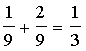5.2 (a)
```
Y2 = Side Bet

-1  1  2  3
--------------
0 | 1  0  0  0 | 1
|            |
1 | 0  1  1  1 | 3
Y1   |            |
2 | 0  2  1  0 | 3
|            |
3 | 0  1  0  0 | 1
|            |
----------------
1  4  2  1 | 8
```

(b) F(2,1) = P[Y1 £ 2, Y2 = 1] =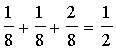5.3 The Sample Space has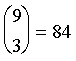elements. Ranges:
Y1 = 0, 1, 2, 3 Number of married executives in the sample
Y2 = 0, 1, 2, 3 Number of never married executives in the sample

To get the joint distribution, f(y1, y2), you have to do a series of "committee selection" problems like those we did in class. For example:

P(Y1=2, Y2=0) = f(y1=2, y2=0) =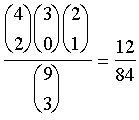and so on.

This produces:
```
Y2

0  1  2  3
--------------
0 | 0  3  6  1 | 10
|            |
1 | 4 24 12  0 | 40
Y1   |            |
2 |12 18  0  0 | 30
|            |
3 | 4  0  0  0 |  4
|            |
----------------
20 45 18  1 | 84
```

5.4 (a)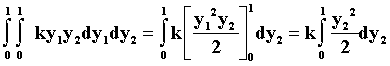= [k(y22)/4]|01 = k/4
Hence, k = 4

(b) F(y1,y2) =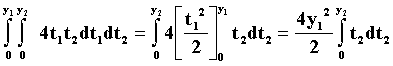=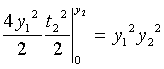Hence: F(y1,y2) =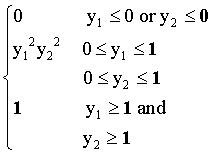(c)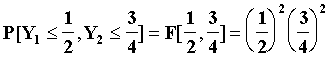=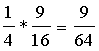5.13 (a) Using results in 5.1

f1(y1) =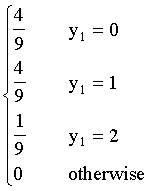(b) No. Note f(y1) =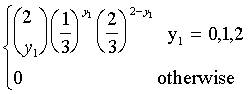Which yields: f(0) =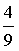, f(1) =, f(2) =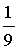5.14 (a) Using the results in 5.2

f2(y2) =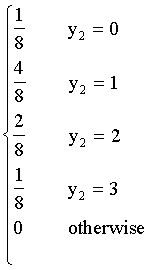(b) P[Y1=3|Y2=1] =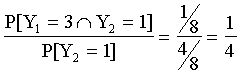5.15 (a) Using results from 5.3
```
{ 10/84  y1 = 0
{
{ 40/84  y1 = 1
{
f(y1) = { 30/84  y1 = 2
{
{  4/84  y1 = 3
{
{ 0 otherwise

```

(b) P(Y1 = 1 | Y2 = 2) = P(Y1 = 1 Ç Y2 = 2)/ P(Y2 = 2) = (12/84)/(18/84)
Using the table shown in 5.3.

(c) P(Y3 = 1 | Y2 = 1) = P(Y3 = 1 Ç Y2 = 1)/ P(Y2 = 1) = (24/84)/(45/84)
Where
```
æ4öæ2öæ3ö
ç ÷ç ÷ç ÷
è1øè1øè1ø
P(Y3 = 1 Ç Y2 = 1) =  --------
æ9ö
ç ÷
è3ø
```
(d) The probabilities are identical.

5.31 Y1 and Y2 are not independent. From the table shown in 5.3 above, it is obvious that:

f(0, 0) ¹ f1(0)f2(0)

5.44 (a) Use E[Y1] = np = 2*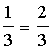(b) Use VAR[Y1] = np(1-p) =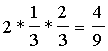(c) E[Y1 - Y2] = E[Y1] - E[Y2] = 0

Because both distributions are identical.

5.45 Using f(y1) from 5.15a:

E(Y1) = 0*(10/84) + 1*(40/84) + 2*(30/84) + 3*(4/84) = 112/84 = 4/3